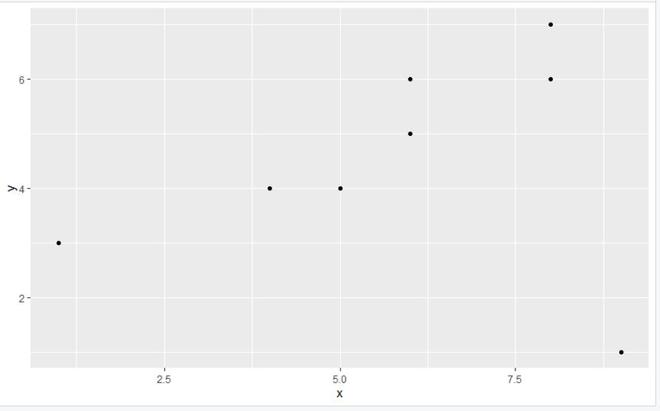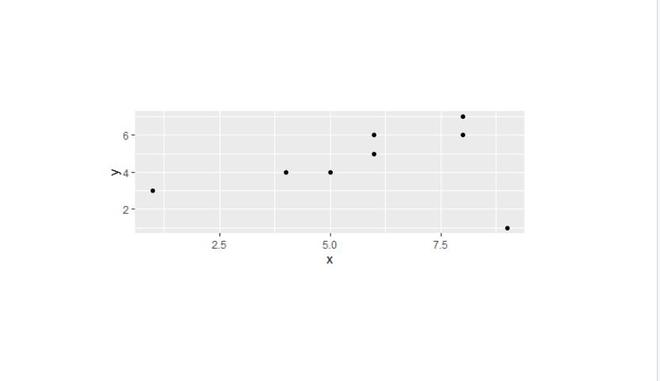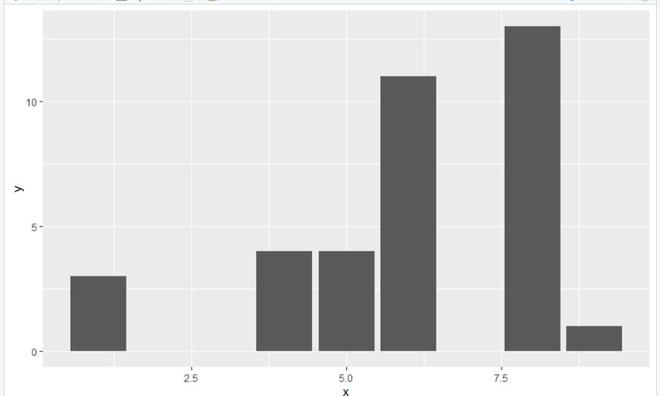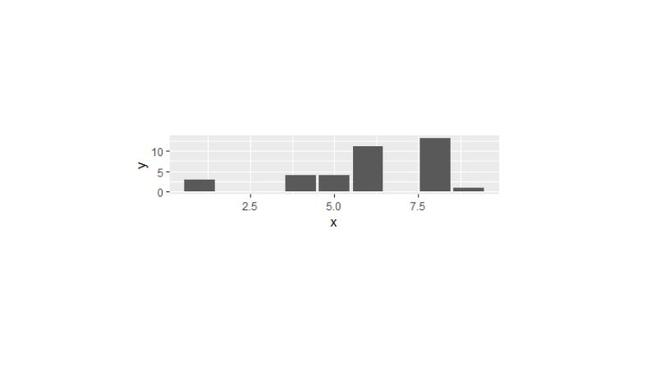Set Area Margins of ggplot2 Plot in R

• Last Updated : 23 Sep, 2021

In this article, we will discuss how to set area margins of the ggplot2 plot in the R programming language.

To do this call the theme() function and use the plot.margin argument of this function with the required data to this argument as per the requirement of the user.

theme() function is a powerful way to customize the non-data components of your plots: i.e. titles, labels, fonts, background, gridlines, and legends.

Syntax:

theme(plot.margin,….)

Parameters:

• plot.margin: -margin around the entire plot (unit with the sizes of the top, right, bottom, and left margins)

Let us look at few implementations to be familiar with the concept

Example 1a: Initial plot

R

 library('ggplot2')  gfg<-data.frame(x=c(8,6,5,1,8,9,6,4),                y=c(6,5,4,3,7,1,6,4))gfg_plot <- ggplot(gfg, aes(x, y)) + geom_point()  gfg_plot

Output:Example 1b: final plot

R

 library('ggplot2')  gfg<-data.frame(x=c(8,6,5,1,8,9,6,4),                y=c(6,5,4,3,7,1,6,4))gfg_plot <- ggplot(gfg, aes(x, y)) + geom_point()gfg_plot+theme(plot.margin = unit(c(4,4,4,4), "cm"))

Output:Example 2a: Initial plot

R

 library('ggplot2')  gfg<-data.frame(x=c(8,6,5,1,8,9,6,4),                y=c(6,5,4,3,7,1,6,4))gfg_plot <- ggplot(gfg, aes(x, y)) +geom_bar(stat="identity")  gfg_plot

Output:Example 2b: final plot

R

 library('ggplot2')    gfg<-data.frame(x=c(8,6,5,1,8,9,6,4),                y=c(6,5,4,3,7,1,6,4))gfg_plot <- ggplot(gfg, aes(x, y)) +geom_bar(stat="identity")  gfg_plot+theme(plot.margin = unit(c(5,5,5,5), "cm"))

Output:My Personal Notes arrow_drop_up